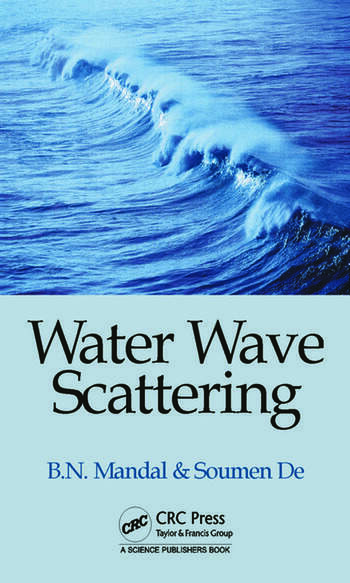Water Wave Scattering

1st Edition

Birendra Nath Mandal, Soumen De

CRC Press
Published May 20, 2015
Reference - 376 Pages - 202 B/W Illustrations
ISBN 9781498705523 - CAT# K24772

For Instructors Request Inspection Copy

USD\$155.00

FREE Standard Shipping!

Preview

Summary

The theory of water waves is most varied and is a fascinating topic. It includes a wide range of natural phenomena in oceans, rivers, and lakes. It is mostly concerned with elucidation of some general aspects of wave motion including the prediction of behaviour of waves in the presence of obstacles of some special configurations that are of interest to ocean engineers. Unfortunately, even the apparently simple problems appear to be difficult to tackle mathematically unless some simplified assumptions are made. Fortunately, one can assume water to be an incompressible, in viscid and homogeneous fluid. The linearised theory of water waves is based on the assumption that the amplitude of the motion is small compared to the wave length. If rotational motion is assumed, then the linearised theory of water waves is essentially concerned with solving the Laplace equation in the water region together with linearised boundary condition. There are varied classes of problems that have been/are being studied mathematically in the literature within the framework of linearised theory of water waves for last many years. Scattering by obstacles of various geometrical configurations is one such class of water wave problems. This book is devoted to advanced mathematical work related to water wave scattering. Emphasis is laid on the mathematical and computational techniques required to study these problems mathematically.

The book contains nine chapters. The first chapter is introductory in nature. It includes the basic equations of linearised theory for a single layer fluid, a two-layer fluid, solution of dispersion equations, and a general idea on scattering problems and the energy identity in water with a free surface. Chapter 2 is concerned with wave scattering involving thin rigid plates of various geometrical configurations, namely, plane vertical barriers or curved barriers, inclined barriers, horizontal barrier, and also thin elastic vertical plate. For the horizontal case, the barrier is submerged below an ice-cover modelled as a thin elastic plate floating on water. Chapter 3 discusses wave scattering by a rectangular trench by using Galerkin technique. Chapter 4 involves wave scattering by a dock by using Carleman singular integral equation followed by reduction to Riemann-Hilbert problems. Chapter 5 involves several wave scattering problems involving discontinuities at the upper surface of water by using the Wiener-Hopf technique, by reduction to Carleman singular integral equations. Chapter 6 considers scattering by a long horizontal circular cylinder either half immersed or completely submerged. In chapter 7, some important energy identities are derived for scattering problems in a single-layer and also in a two-layer fluid. Chapter 8 is concerned with wave scattering in a two-layer fluid by a thin vertical plate and by a long horizontal circular cylinder submerged in either of the two layers. Chapter 9 is the final chapter which considers a number of wave scattering problems in a single-layer or a two-layer fluid with variable bottom topography by using a simplified perturbation analysis

It is hoped that this book will be useful to researchers on water waves. The several wave scattering problems presented in the book are mostly based on the research work carried out by the authors and their associates.

Instructors

We provide complimentary e-inspection copies of primary textbooks to instructors considering our books for course adoption.